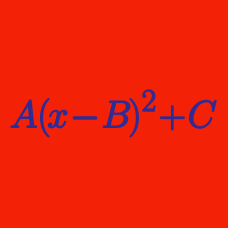Algebra

# Completing the Square - Multiple Variables

For real numbers $x$, $y$ and $z$, function $f(x,y,z)=4x-x^2-y^2-z^2+18$ has a maximum value $d$ at $x=a$, $y=b$ and $z=c$. What is the value of $a+b+c+d$?

For real numbers $x$, $y$ and $z$, function $f(x,y,z)=x^2+y^2+z^2-4x-4y-4z+14$ has a minimum value $d$ at $x=a$, $y=b$ and $z=c$. What is the value of $a+b+c+d$?

For real numbers $x$ and $y$, function $f(x,y)=x^2+2y^2-4x-16y+21$ has a minimum value $c$ at $x=a$ and $y=b$. What is the value of $a+b-c$?

For real numbers $x$ and $y$, function $f(x,y)=2x-x^2+2y-y^2+28$ has a maximum value $c$ at $x=a$ and $y=b$. What is the value of $a+b+c$?

What is the maximum value of $f(f(x))$ in the domain $2 \leq x \leq 5$ for the function $f(x)=x^2-6x+4?$

×